Examples

Chapter 9 Class 12 Differential Equations
Serial order wise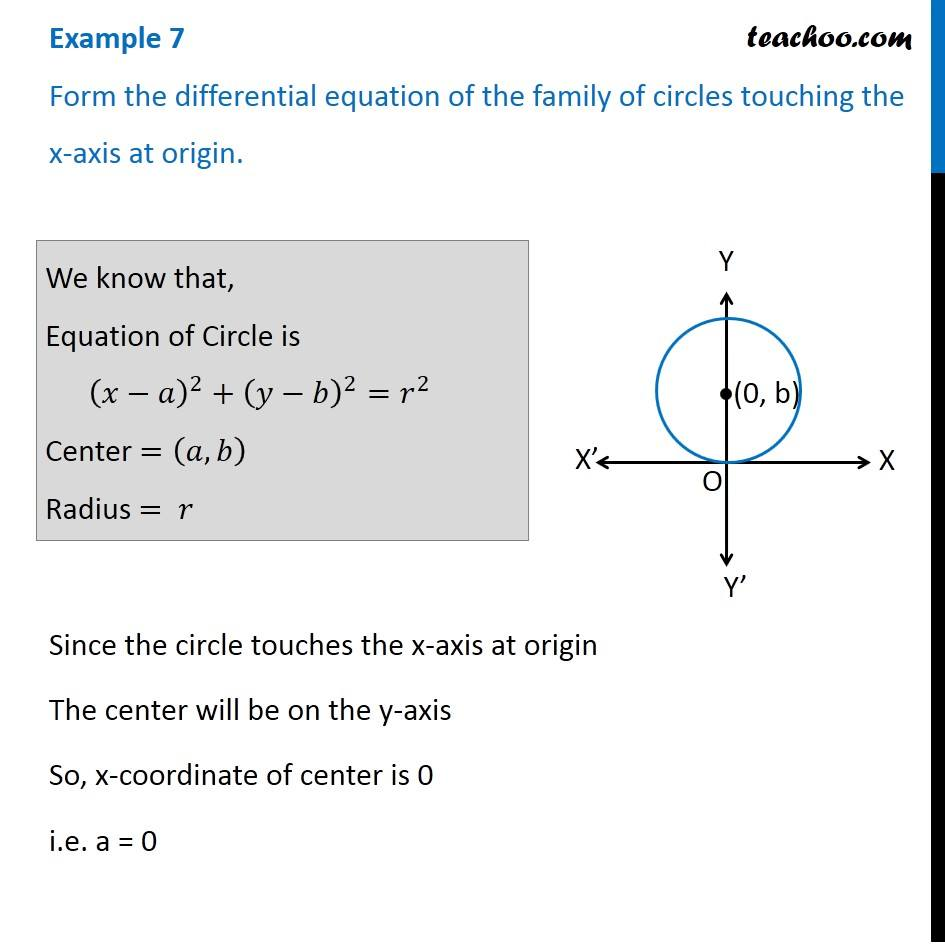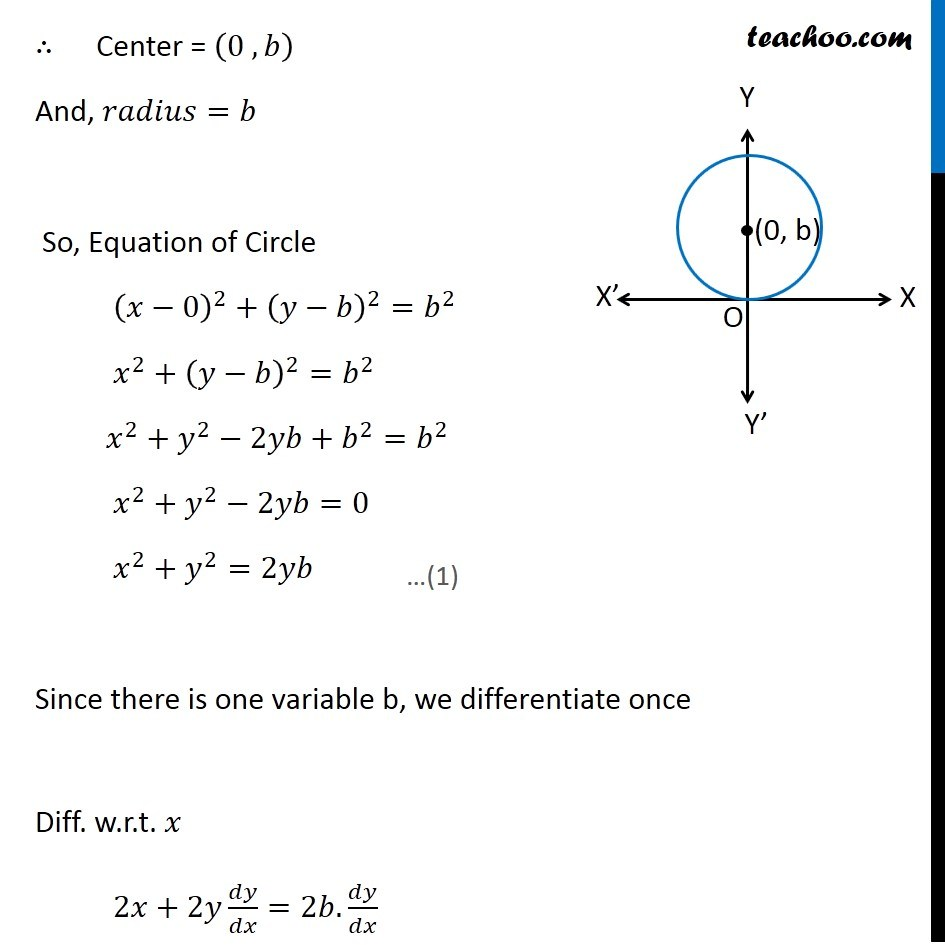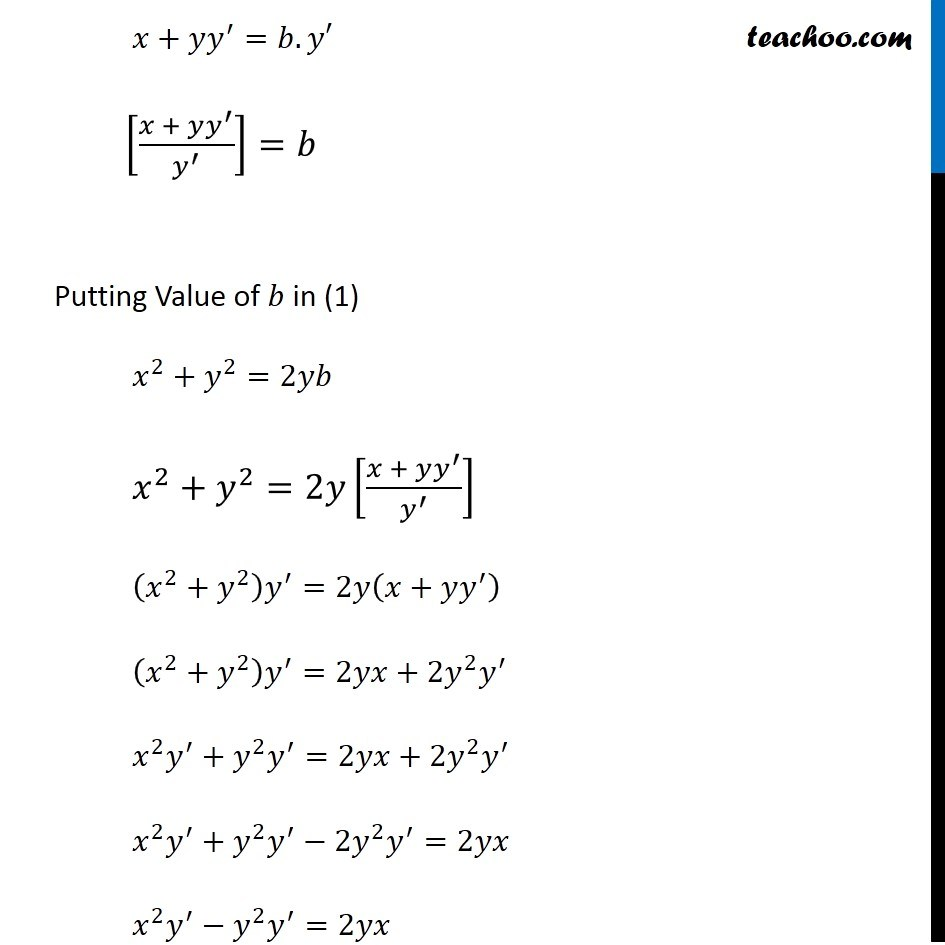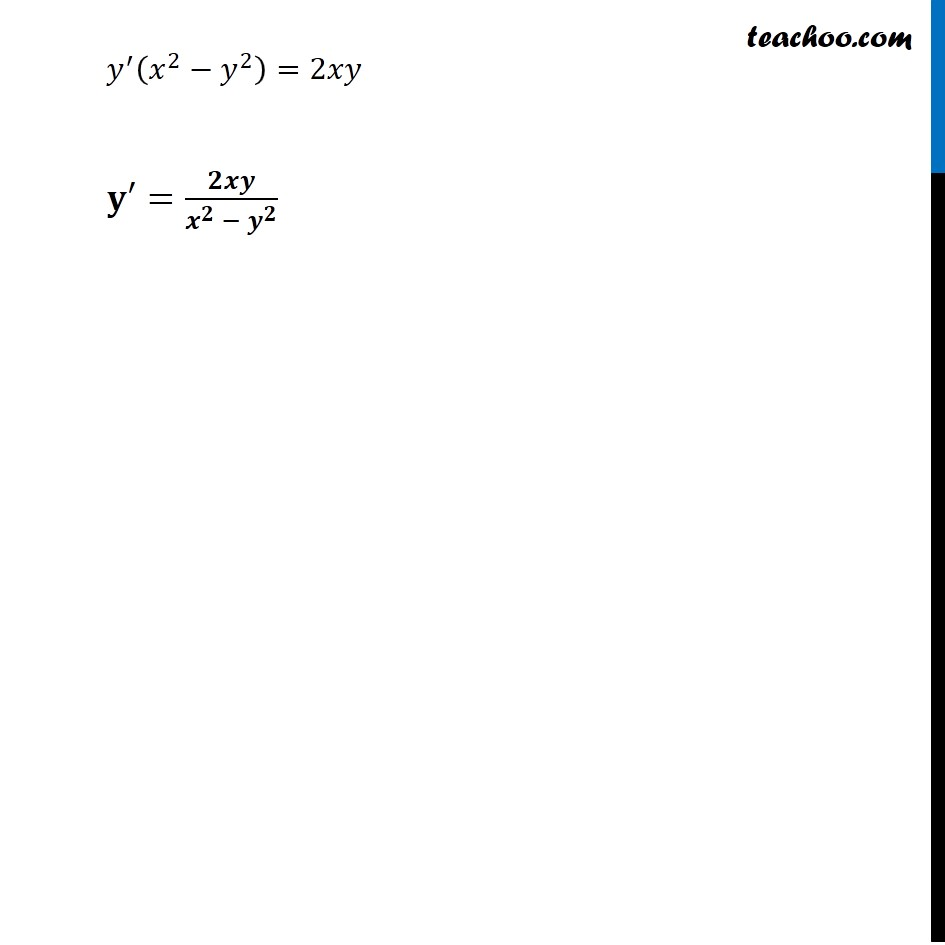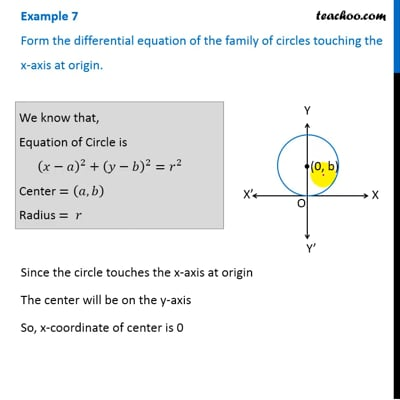This video is only available for Teachoo black users

Maths Crash Course - Live lectures + all videos + Real time Doubt solving!

### Transcript

Example 7 Form the differential equation of the family of circles touching the x-axis at origin. We know that, Equation of Circle is (𝑥−𝑎)^2+(𝑦−𝑏)^2=𝑟^2 Center =(𝑎,𝑏) Radius = 𝑟 Since the circle touches the x-axis at origin The center will be on the y-axis So, x-coordinate of center is 0 i.e. a = 0 ∴ Center = (0 , 𝑏) And, 𝑟𝑎𝑑𝑖𝑢𝑠=𝑏 So, Equation of Circle (𝑥−0)^2+(𝑦−𝑏)^2=𝑏^2 𝑥^2+(𝑦−𝑏)^2=𝑏^2 𝑥^2+𝑦^2−2𝑦𝑏+𝑏^2=𝑏^2 𝑥^2+𝑦^2−2𝑦𝑏=0 𝑥^2+𝑦^2=2𝑦𝑏 Since there is one variable b, we differentiate once Diff. w.r.t. 𝑥 2𝑥+2𝑦 𝑑𝑦/𝑑𝑥=2𝑏.𝑑𝑦/𝑑𝑥 𝑥+𝑦𝑦^′=𝑏.𝑦′ [(𝑥 + 𝑦𝑦^′)/𝑦^′ ]=𝑏 Putting Value of 𝑏 in (1) 𝑥^2+𝑦^2=2𝑦[(𝑥 + 𝑦𝑦^′)/𝑦^′ ] (𝑥^2+𝑦^2 ) 𝑦^′=2𝑦(𝑥+𝑦𝑦^′ ) (𝑥^2+𝑦^2 ) 𝑦^′=2𝑦𝑥+2𝑦^2 𝑦^′ 𝑥^2 𝑦^′+𝑦^2 𝑦^′=2𝑦𝑥+2𝑦^2 𝑦^′ 𝑥^2 𝑦^′+𝑦^2 𝑦^′−2𝑦^2 𝑦^′=2𝑦𝑥 𝑥^2 𝑦^′−𝑦^2 𝑦^′=2𝑦𝑥 𝑦^′ (𝑥^2−𝑦^2 )=2𝑥𝑦 𝐲′=𝟐𝒙𝒚/(𝒙^𝟐 − 𝒚^𝟐 )